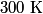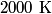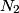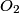## Solution to 1986 Problem 87

 The vibrational degrees of freedom for a diatomic molecule are dead at$300 \mbox{ K}$ (room temperature), while the rotational degrees of freedom are active at$300 \mbox{ K}$. When the temperature is increased beyond$2000 \mbox{ K}$, the vibrational degrees of freedom become active for$N_2$ (nitrogen) and$O_2$ (oxygen). Thus, answer (E) is correct.